# Chauncey

Chauncey is building a storage bench for his son’s playroom. The storage bench will fit into the corner and against two walls to form a triangle. Chanuncy wants to buy a triangular shaped cover for the bench.

If the storage bench is 2 1/2 ft. Along one wall and 4 1/4 ft. Along the other wall, how big will the cover have to be to cover the entire bench?

Correct result:

A =  5.3125 ft2

#### Solution: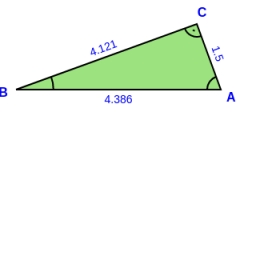We would be pleased if you find an error in the word problem, spelling mistakes, or inaccuracies and send it to us. Thank you!Tips to related online calculators

#### You need to know the following knowledge to solve this word math problem:

We encourage you to watch this tutorial video on this math problem:

## Next similar math problems:

• Here isHere is a data set (n=117) that has been sorted. 10.4 12.2 14.3 15.3 17.1 17.8 18 18.6 19.1 19.9 19.9 20.3 20.6 20.7 20.7 21.2 21.3 22 22.1 22.3 22.8 23 23 23.1 23.5 24.1 24.1 24.4 24.5 24.8 24.9 25.4 25.4 25.5 25.7 25.9 26 26.1 26.2 26.7 26.8 27.5 27.6 2
• Dusana) Dusan break two same window, which has triangular shape with a length of 0.8 m and corresponding height 9.5 dm. Find how many dm2 of glass he needs to buy for glazing of these windows. b) Since the money to fix Dusan has not, must go to the paint job
• Top of the towerThe top of the tower has the shape of a regular hexagonal pyramid. The base edge has a length of 1.2 m, the pyramid height is 1.6 m. How many square meters of sheet metal is needed to cover the top of the tower if 15% extra sheet metal is needed for joint
• Four prismsQuestion No. 1: The prism has the dimensions a = 2.5 cm, b = 100 mm, c = 12 cm. What is its volume? a) 3000 cm2 b) 300 cm2 c) 3000 cm3 d) 300 cm3 Question No.2: The base of the prism is a rhombus with a side length of 30 cm and a height of 27 cm. The heig
• Tiles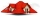The pupils of the building school have to calculate how many roof tiles they will need to cover the roof of the house in the shape of two rectangles measuring 10 x 7.2 m. One roof tile covers a rectangular area of 22 cmx32 cm. How many tiles will they nee
• Chocolate rollThe cube of 5 cm chocolate roll weighs 30 g. How many calories will contain the same chocolate roller of a prism shape with a length of 0.5 m whose cross section is an isosceles trapezoid with bases 25 and 13 cm and legs 10 cm. You know that 100 g of this
• Paper box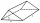Calculate how much we'll pay for a three-side shaped prism box with a triangular base, and if it measures 12cm and 1.6dm, the hypotenuse measures 200mm. The box is 34cm high. We pay 0,13 € per square meter of paper.
• GarageThere are two laths in the garage opposite one another: one 2 meters long and the second 3 meters long. They fall against each other and stay against the opposite walls of the garage and both laths cross 70 cm above the garage floor. How wide is the garag
• Octagon from rectangleFrom tablecloth rectangular shape with dimensions of 4 dm and 8 dm we cuts down the corners in the shape of isosceles triangles. It thus formed an octagon with area 26 dm2. How many dm2 we cuts down?
• Mouse Hryzka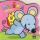Mouse Hryzka found 27 identical cubes of cheese. She first put in a large cube out of them and then waited for a while before the cheese cubes stuck together. Then from every wall of the big cube she will eats the middle cube. Then she also eats the cube
• Ethernet cableCharles and George are passionate gamers and live in houses that are exactly opposite each other across the street, so they can see each other through the windows. They decided that their computers will connect the telephone cable in order to play games t
• Laths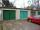There are two laths in the garage opposite one another: one 2 meters long and the other 3 meters long. They fall against each other and lean against the opposite walls of the garage both laths and touch at a height of 70 cm above the garage floor. How wid
• Right triangular prismWe have cuboid with a base and dimensions of 12 cm and 5 cm and height of 4 cm. The tablecloth cut it into two identical triangular prisms with right triangular bases. The surface of the created prisms was painted with color. Calculate the surface area of
• The gardenThe garden has the shape of a rectangular trapezium. The bases have lengths of 27 meters and 36 meters, the trapezoid's height is 12 meters. Calculate how much fence will cost this garden if one meter costs 1.5 €?
• Rectangular trapezoidThe ABCD rectangular trapezoid with the AB and CD bases is divided by the diagonal AC into two equilateral rectangular triangles. The length of the diagonal AC is 62cm. Calculate trapezium area in cm square and calculate how many differs perimeters of the
• Jared's room paintingJared wants to paint his room. The dimensions of the room are 12 feet by 15 feet, and the walls are 9 feet high. There are two windows that measure 6 feet by 5 feet each. There are two doors, whose dimensions are 30 inches by 6 feet each. If a gallon of p
• The tentThe tent shape of a regular quadrilateral pyramid has a base edge length a = 2 m and a height v = 1.8 m. How many m2 of cloth we need to make the tent if we have to add 7% of the seams? How many m3 of air will be in the tent?